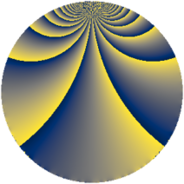# Properties

 Label 1152.1.tLevel $1152$ Weight $1$ Character orbit 1152.t Rep. character $\chi_{1152}(319,\cdot)$ Character field $\Q(\zeta_{6})$ Dimension $4$ Newform subspaces $1$ Sturm bound $192$ Trace bound $0$

# Related objects

## Defining parameters

 Level: $$N$$ $$=$$ $$1152 = 2^{7} \cdot 3^{2}$$ Weight: $$k$$ $$=$$ $$1$$ Character orbit: $$[\chi]$$ $$=$$ 1152.t (of order $$6$$ and degree $$2$$) Character conductor: $$\operatorname{cond}(\chi)$$ $$=$$ $$72$$ Character field: $$\Q(\zeta_{6})$$ Newform subspaces: $$1$$ Sturm bound: $$192$$ Trace bound: $$0$$

## Dimensions

The following table gives the dimensions of various subspaces of $$M_{1}(1152, [\chi])$$.

Total New Old
Modular forms 52 4 48
Cusp forms 20 4 16
Eisenstein series 32 0 32

The following table gives the dimensions of subspaces with specified projective image type.

$$D_n$$ $$A_4$$ $$S_4$$ $$A_5$$
Dimension 4 0 0 0

## Trace form

 $$4q + 2q^{9} + O(q^{10})$$ $$4q + 2q^{9} + 4q^{17} - 2q^{25} + 6q^{33} + 2q^{41} - 2q^{49} - 6q^{57} + 4q^{73} - 2q^{81} - 8q^{89} - 2q^{97} + O(q^{100})$$

## Decomposition of $$S_{1}^{\mathrm{new}}(1152, [\chi])$$ into newform subspaces

Label Dim. $$A$$ Field Image CM RM Traces $q$-expansion
$$a_2$$ $$a_3$$ $$a_5$$ $$a_7$$
1152.1.t.a $$4$$ $$0.575$$ $$\Q(\zeta_{12})$$ $$D_{6}$$ $$\Q(\sqrt{-2})$$ None $$0$$ $$0$$ $$0$$ $$0$$ $$q-\zeta_{12}q^{3}+\zeta_{12}^{2}q^{9}+(\zeta_{12}^{3}+\zeta_{12}^{5}+\cdots)q^{11}+\cdots$$

## Decomposition of $$S_{1}^{\mathrm{old}}(1152, [\chi])$$ into lower level spaces

$$S_{1}^{\mathrm{old}}(1152, [\chi]) \cong$$ $$S_{1}^{\mathrm{new}}(72, [\chi])$$$$^{\oplus 5}$$$$\oplus$$$$S_{1}^{\mathrm{new}}(288, [\chi])$$$$^{\oplus 3}$$